## 4.15Inlet-outlet-velocity condition

The inlet-outlet condition was described in Sec. 4.10 , which switches between the zero gradient type for outﬂow and ﬁxed value for inﬂow. The condition is not so suitable for velocity, e.g. at a free boundary, since it is requires an inlet velocity to be prescribed when inﬂow occurs.

The switching based on the ﬂow direction is not a problem in itself. The ﬂow direction comes from the sign of the ﬂux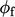, as noted in Eq. (4.12 ), which is determined by the solution of the pressure equation (part of pressure-velocity coupling described in Chapter 5 ).

The problem is instead that a value of velocity cannot be chosen in the case of inﬂow since the inﬂow speed is determined by the solution within the domain. This suggests a zero gradient type as a more suitable condition.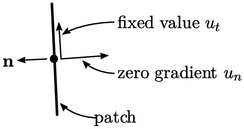However, Sec. 4.3 discusses that all but one variable should be prescribed at a boundary with inﬂow. For velocity that variable could be its normal component, while a ﬁxed value could still be applied to the tangential component.

The direction mixed type described in Sec. 4.14 provides the framework to implement this condition. A reference value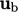is speciﬁed and the reference gradient is zero, i.e.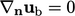.

The value fraction tensor is calculated as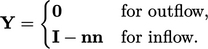(4.26)
The ﬁxed value which is applied must be a velocity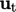tangential to the boundary, which can be calculated by subtracting the normal component from a speciﬁed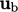by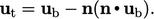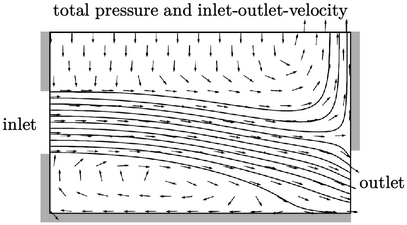This inlet-outlet-velocity condition can be applied at the free boundary in the example from Sec. 4.6 . For free boundaries like this,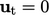is the only practical speciﬁcation, which causes all inﬂow to be normal to the free boundary, as shown above.

The solution is clearly diﬀerent to that using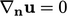shown on page 267 . There, the inﬂow direction is determined by the solution rather than prescribed. The solution with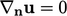may be more accurate but the inlet-outlet-velocity condition adheres better to the general principles of boundary conditions, so is likely to be more stable.

It becomes less contentious to setwith the inlet-outlet-velocity condition as the boundary is moved further into the far ﬁeld, where the ﬂow is more quiescent.

Notes on CFD: General Principles - 4.15 Inlet-outlet-velocity condition